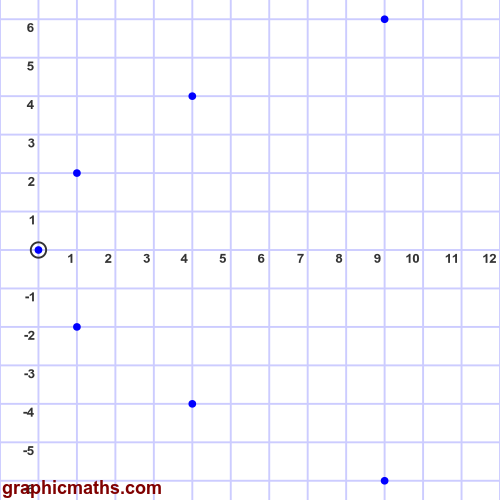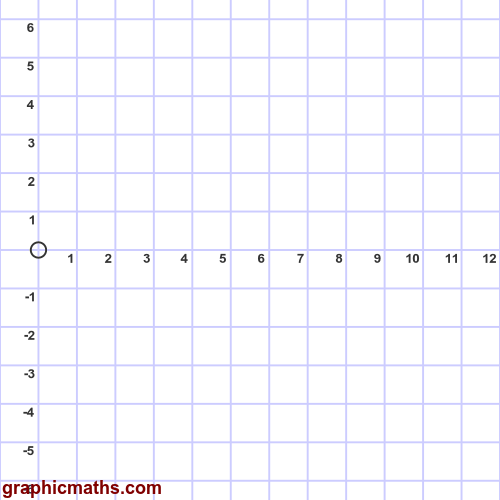# Parabola example

Martin McBride, 2020-09-12
Tags parametric equation parabola
Categories coordinate systems pure mathematics

A parabola is a curve with the parametric equations:

\begin{align} x = a t^2\newline y = 2 a t \end{align}

Where $a$ is a positive constant, and $t$ is the independent variable.

We can plot this curve by calculating the values of $x$ and $y$ for various values of $t$, and drawing a smooth curve through them.

## Curve for a = 1

Assuming $a = 1$, the parametric equations simplify to:

\begin{align} x = t^2\newline y = 2 t \end{align}

The values are shown in the following table, for $t$ in the range -3 to +3:

t x y
-3 9 -6
-2 4 -4
-1 1 -2
0 0 0
1 1 2
2 4 4
3 9 6

Here are the points plotted on a graph:The curve can be drawn by plotting the points and drawing a smooth line through them: